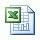## Increase Mud Weight by Adding Calcium Carbonate

Carbonate is weighing agent mostly used in horizontal well because it does not plug up formation. We can use the same weight up concept with barite to calculate how to weight up with carbonate. Follow a topic below to learn how to weight up with calcium carbonate.

NOTE: The maximum practical mud weight attainable with calcium carbonate is 14.0 ppg.

This formula below is used for calculating how many sacks of calcium carbonate required per 100 bbl of drilling fluid (Mud weight increase with calcium carbonate (SG – 2.7))

Sacks of calcium carbonate per 100 bbl of mud = 945 x (W2 – W1) ÷ (22.5 – W2)

Where; W1 = current mud weight in ppg

W2 = new mud weight in ppg

Example: Determine the number of sacks of calcium carbonate per l00 bbl required to increase the density from 10.0 ppg (W1) to 13.0 ppg (W2):
Sacks of calcium carbonate per 100 bbl of mud = 945 x(13.0 – 10.0) ÷ (22.5 – 13.0)

Sacks of calcium carbonate per 100 bbl of mud = 298.4 sacks
If you have total volume of 500 bbl of mud, calcium carbonate required to increase mud weight from 10.0 ppg to 13.0 ppg is 1,492 sacks (298.4×500/100).

Please find theExcel sheet for calculating how many sacks of calcium carbonate required per 100 bbl of drilling fluid.

## Starting volume of original mud (weight up with Barite)

You know how much volume will be increased due to adding barite into the system; however, you sometimes are limited to total volume due to limit pit volume on the rig so you need to calculate starting volume to achieve the predetermined final volume of desired mud weight. This formula below is used to determine the star volume of mud in case of weighting up with Barite.

Starting volume in bbl = VF x (35 – W2) ÷ (35 – W1)

Where; W1 = current mud weigth in ppg

W2 = new mud weight in ppg

VF = final volume in bbl

Example: Determine the starting volume (bbl) of 10.0 ppg (W1) mud required to achieve 100 bbl (VF) of 13.0 ppg (W2) mud with barite:

Starting volume in bbl = 100 x (35 – 13.0) ÷ (35 – 10.0)

Starting volume in bbl= 88.0 bbl

In order to achieve 100 bbl of 13.0 ppg mud weight up with barite, you must have 88.0 bbl starting volume of 10.0 ppg mud.

Please find the Excel sheetfor calculating Barrel of starting volume of original mud weight required to give a predetermined final volume of desired mud weight with barite.

## Volume of mud in bbl increase due to adding bariteAfter adding barite to increase mud weight in the system, total mud volume will increase due to volume of dry barite. This formula below shows you how to determine volume of mud increase in bbl per 100 bbl of original mud.

Volume increase per 100 bbl of mud = 100 x (W2 – W1) ÷ (35 – W2)

Where;

W1 = current mud weight
W2 = new mud weight

Example: Determine the volume increase when increasing the density with barite from 10.0 ppg (W1) to 13.0 ppg (W2):

Volume increase per 100 bbl of mud = 100 x (13.0 – 10.0) ÷ (35 – 13.0)

Volume increase per 100 bbl of mud = 13.64 bbl

If you have total volume of 500 bbl of mud, the volume increase due to adding barite will be equal to 68.2 bbl (13.64 x 500 ÷ 100).

Please find the Excel sheet for calculating volume of mud in bbl increase due to adding Barite.

Ref books: Lapeyrouse, N.J., 2002. Formulas and calculations for drilling, production and workover, Boston: Gulf Professional publishing.

Bourgoyne, A.J.T., Chenevert , M.E. & Millheim, K.K., 1986. SPE Textbook Series, Volume 2: Applied Drilling Engineering, Society of Petroleum Engineers.

Mitchell, R.F., Miska, S. & Aadny, B.S., 2011. Fundamentals of drilling engineering, Richardson, TX: Society of Petroleum Engineers.

## Increase mud weight by adding bariteWeighting up is the critical operation while drilling so we need to know how much weighting agent such as barite, calcium carbonate, hematite, etc required in order to get proper weight to prevent a well control situation. This mud calculation is separated into several sub topics so you can follow each one easily. For this article, it will demonstrate how to calculate how many sacks of barite needed to increase mud weight in ppg with barite. (average specific gravity of barite – 4.2)

The formula for weight up with barite is listed below:

Sacks of Barite per 100 bbl of mud = 1470 x (W2 – W1) ÷ (35 – W2)

Where; W1 = current mud weight

W2 = new mud weight

Example: Determine the number of sacks of barite required to increase the density of

500 bbl of 10.0 ppg (W1) mud to 13.0 ppg (W2):

Sacks of Barite per 100 bbl of mud = 1470 x (13.0 – 10.0) ÷ (35 – 13.0)

Sacks of Barite per 100 bbl of mud = 201 sacks (round up)

If you have total volume of 500 bbl of mud, the barite required to increase mud weight from 10.0 ppg to 13.0 ppg is 1,003 sacks (201×500/100).

Please find the Excel sheet used for weighting up with Barite.

Ref books: Lapeyrouse, N.J., 2002. Formulas and calculations for drilling, production and workover, Boston: Gulf Professional publishing.

Bourgoyne, A.J.T., Chenevert , M.E. & Millheim, K.K., 1986. SPE Textbook Series, Volume 2: Applied Drilling Engineering, Society of Petroleum Engineers.

Mitchell, R.F., Miska, S. & Aadny, B.S., 2011. Fundamentals of drilling engineering, Richardson, TX: Society of Petroleum Engineers.